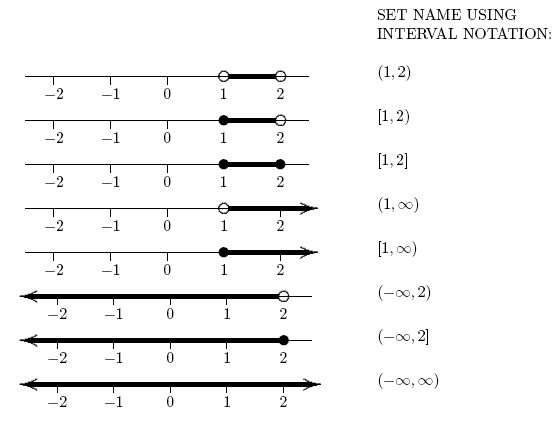﻿ Introduction to Sets
INTRODUCTION TO SETS
by Dr. Carol JVF Burns (website creator)
Follow along with the highlighted text while you listen!
Keep in mind:
Math is primarily designed as a written language, not a spoken language!
Some things are read aloud in a simple (but slightly incomplete) way,
assuming that you're looking at the math while it is being read.
• PRACTICE (online exercises and printable worksheets)
Want more details, more exercises?

DEFINITION set
A set is a collection with the following property:
given any object, either the object is in the collection, or isn't in the collection.
EXAMPLES:
Question: Is ‘the collection of some people’ a set?
Solution: ‘The collection of some people’ is not a set;  it is too vague.
Is your teacher in this collection? Maybe or maybe not!
Question: Is the collection of numbers consisting of $\;3,6,9,12,\ldots\;$ a set?
Solution: Yes.
Is the number (say) $\,35{,}983{,}205{,}119{,}780{,}238{,}482{,}108{,}222\,$ in this collection?
Well, either it is (if divisible by $\,3\,$) or isn't (if not divisible by $\,3\,$).
Notice that it's not important whether you personally know whether the answer is YES or NO;
all that matters is that the answer is definitely YES or NO.

• A set is a mathematical expression.
• Sets can have different names!

DEFINITIONS elements, members;   finite set;   infinite set
The objects in a set are called its elements or its members.

If a set has $\,n\,$ members, where $\,n\,$ is a whole number, then it is called a finite set
(pronounced with a long i, FIGH-night).

If a set is not finite, then it is infinite (pronounced with a short i, IN-fi-nit).
EXAMPLES:
$\{4,10\}\,$ is a finite set, with two members.
The number $\,4\,$ is a member.
The number $\,10\,$ is a member.
(More on list notation for sets below.)
The set $\,\{1,2,3,\ldots\}\,$ is an infinite set.
The number $\,7\,$ is an element of the set.
The number $\,\frac{30}{2}\,$ is an element of the set. (The name we use doesn't matter!)
The number $\,0.25\,$ is not an element of the set.
SYMBOLS USED IN CONNECTION WITH SETS:

The following symbols are used in connection with sets:

• $\cssId{s50}{\{}\;\;\cssId{s51}{\}}\;$ are called braces.
They are used in list notation for sets (see below).
• $\cssId{s54}{(}\;\;\cssId{s55}{)}\;$ are called parentheses. (Singular form is parenthesis.)
They are used in interval notation for sets (see below).
• $\cssId{s59}{[}\;\;\cssId{s60}{]}\;$ are called brackets.
They are used in interval notation for sets (see below).

LIST NOTATION FOR SETS:

• List notation for sets is used whenever the elements of a set can be listed.
• Braces $\;\{\;\;\}\;$ are used for list notation.
• Separate members of the set with commas.
• Use three dots $\;\ldots\;$ to indicate that a pattern is to be repeated.
Be sure to list enough elements to clearly establish the pattern.
• For a finite set, the order that elements are listed doesn't matter.

EXAMPLES:
Here are six names for the same finite set:
$\{1,2,3\}$    or    $\{1,3,2\}$    or    $\{2,1,3\}$    or    $\{2,3,1\}$    or    $\{3,1,2\}$    or    $\{3,2,1\}$
The infinite set $\;\{0,1,2,3,\ldots\}\;$ contains all the whole numbers.
The finite set $\;\{0,1,2,\ldots,1000\}\;$ contains all the whole numbers between $\,0\,$ and $\,1000\,$.
The infinite set $\{2,1,0,\ldots\}\;$ contains all the integers that are less than or equal to $\;2\;$.
These are very different sets!
MEMBERSHIP IN A SET:

• The verb $\;\in\;$ is used to denote membership in a set.
• The sentence $\;x\in S\;$ is read as:
$\,x\,$ is in $\,S\;$’   or   ‘$\,x\,$ is an element of $\,S\;$’   or   ‘$\,x\,$ is a member of $\,S\;$’.
• The sentence $\;x\notin S\;$ is read as:
$\,x\,$ is not in $\,S\;$’   or   ‘$\,x\,$ is not an element of $\,S\;$’   or   ‘$\,x\,$ is not a member of $\,S\;$’.

EXAMPLE:
If $\,S\,$ is the set $\,\{1,2,3\}\,$, then all of the following sentences are true:

$\,1\in S\,$   and   $\,3\in S\,$   and   $\,4\notin S\,$   and   $\,\frac{8}{4}\in S\,$   and   $\,7-4\in S\,$
A SPECIAL SENTENCE:

The sentence ‘ Let $\,S = \{1,2,3\}\,$’ is used to  assign  the name $\,S\,$ to the set $\,\{1,2,3\}\,$.
The word  ‘let’  is the key!

EXAMPLE:
Question: How would a mathematician say:
“Take the set $\,\{a,b,c,d\}\,$ and give it the name $\,T\;$”?
Solution: Let $\,T = \{a,b,c,d\}\;$.
(The word  ‘let’  is a vital part of this sentence!)
DEFINITION empty set
The empty set is the unique set that has no members.
The empty set is denoted using either of these symbols: $\;\emptyset\;$ or $\;\{\;\}$

So, the empty set is empty! It has no members!

Be careful: the set $\,\{\emptyset\}\;$ is a set with one member—it is NOT the empty set.

INTERVAL NOTATION:

• Interval notation is used to describe intervals of real numbers.
• Intervals of real numbers are infinite sets.
• Parentheses $\;(\;\;)\;$ are used when an endpoint is not included.
• Brackets $\;[\;\;]\;$ are used when an endpoint is included.
• The ‘infinity’ symbol $\,\infty\,$ is used to denote that an interval extends forever to the right.
• The ‘negative infinity’ symbol $\,-\infty\,$ is used to denote that an interval extends forever to the left.
• The numbers used in interval notation always go from left to right on the number line.SUBSETS:

Roughly, a subcollection from a set is called a subset.

EXAMPLE:
Let $\,S = \{a,b\}\,$.
Then:
• $\,\{a\}\,$ is a subset (choose only the ‘$\,a\,$’ )
• $\,\{b\}\,$ is a subset (choose only the ‘$\,b\,$’ )
• $\,\{a,b\}\,$ is a subset (choose everything!)
• $\,\{\;\}\,$ is a subset (choose nothing!)
DEFINITION subset
Let $\,S\,$ be a set.
Set $\,B\,$ is called a subset of $\,S\,$ if any one of the following three conditions holds:
• $\,B\,$ is the set $\,S\,$ itself
• $\,B\,$ is the empty set
• each member of $\,B\,$ is also a member of $\,S\,$
Master the ideas from this section